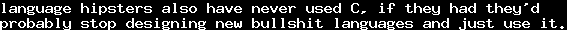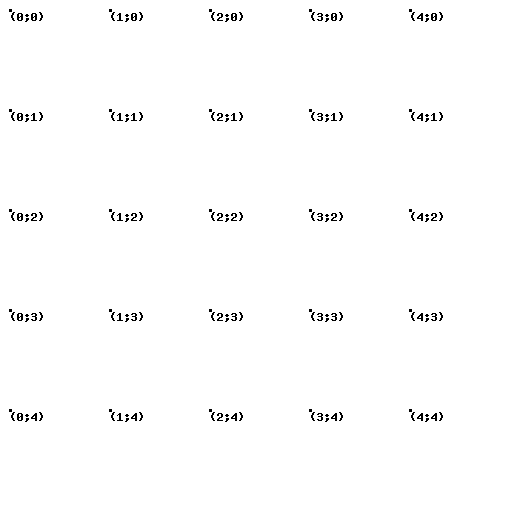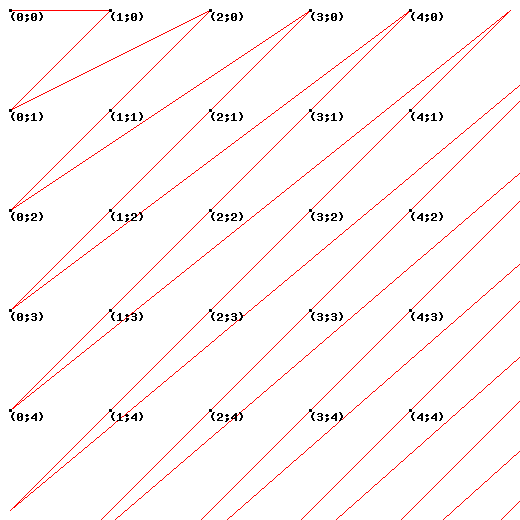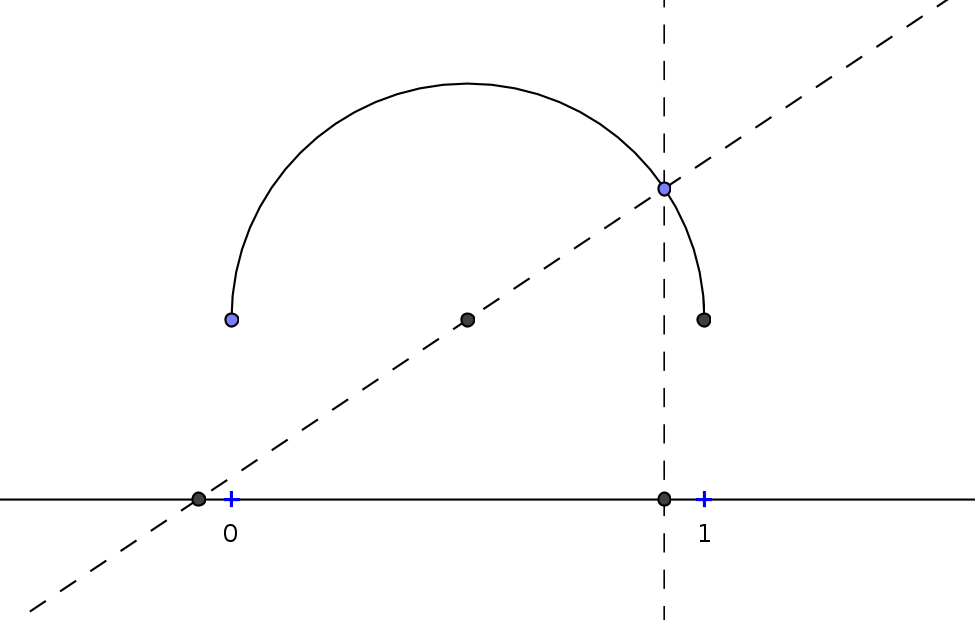# On Infinity 4 - Countable SetsTue, 17 Jan 2012 20:15:00 GMT

We have seen a basic introduction to infinity and its definition in part 1 of this series, and in part 2, we have seen that infinitary processes might have a finite outcome. In part 3, we have seen a lot of nice examples getting deeper into the topic of infinite curves and planes. However, we moved away from the actual question about how many elements a set has, as the curves are more demonstrative in the beginning. With this post, we want to turn back to this question again.

Let us recall how we defined infinity in part 1: A set is infinite if and only if there is a bijection into a proper subset.

While it is pretty clear that there are multiple finite sets with different numbers of elements, the question whether there are infinite sets that contain more elements thanhas not been answered yet. And while it is pretty clear that for two finite sets, we can always say which of them is greater, this is not clear when talking about infinite sets. The definition is pretty obvious: If there is a bijection from a setinto a subset of, thenhas more or equally many elements as, we will denote this by.
So as said, the first question which arises is, whether two sets are always comparable. This follows directly from the well-ordering theorem, but we will not discuss this proof here, since it is sophisticated. We just keep in mind that for any two setsand, we haveor.

Let us denote bythat the setsandcontain equally many elements, that is, there is a bijection between them. Then the obvious question is whetherandimplies. Do not let yourself confuse by the notation: This is not clear! Essentially, the question is whether when we have a bijection fromto a subset ofand a bijection frominto a subset of, we also have a bijection betweenand.
By the Cantor-Bernstein-Theorem, this holds, but we will not discuss this here, and just accept that it works for all sets we will consider.

Now, when thinking about the "number" of Elements of the setof positive integers, two obvious questions arise: Whether there is an infinite set smaller than, and whether there is an infinite set greater than. For the first question, it is sufficient to prove that every infinite subset ofhas a bijection into. This can be done by the minimum principle defined in part 1, and is left to the interested reader. So indeed,is the "smallest" infinite set, in the sense that every other infinite set has at least as much elements as.

In part 1, we have already seen, that the first obvious "larger" set, the setof integers, is not larger than: The mapping, that is,is, is a bijection. So in fact, there are as many non-negative integers, as there are integers at all.

The next obvious candidate is the setof pairsof nonnegative integers. You can imagine them as points of a "grid":The question can now be seen more geometrical: Is it possible, to arrange these points on a curve? And of course, it is:We get the sequence. This sequence is defined by the Cantor-Pairing-Function. It is a bijection, and we call its inverse for the right sideand its inverse for the left side, thus,.

So,is not larger than. In fact, therefore, trivially, also the set of triples, quartuples, etc., of natural numbers are not larger than the set of natural numbers, as we can encode for example triples by.

So, the next obvious candidate would be to unite all of these sets, gaining the setof finite sequences of natural numbers. As you might expect, this set is also countable, and there is a more general theorem that states that every union of countably many countable sets is countable itself, which would hold here. But in this case, we can show it more directly, by giving a bijection. Firstly, letbe the bijection from the-tuples into the natural numbers (where), which exists as shown above. Then defineIts inverse is given byAs this looks rather theoretic and confusing, here is a Java-Program which actually does this (I hope):

public class CantorStuff {    public static int cantorPair (int x, int y) {    x--; y--;    return (x+y)*(x+y+1)/2+y+1;    }    public static int[] cantorPairToNumbers (int z) {    z--;    int w = (int) Math.floor((Math.sqrt(8*z+1)-1)/2);    int t = (w*w+w)/2;    int y = z-t;    return new int [] { w-y+1, y+1 };    }    public static int sequenceToNum (int[] seq) {    int ret = seq[seq.length - 1];    for (int i = seq.length - 2; i >= 0; i--) {        ret = cantorPair(seq[i], ret);    }    return cantorPair(seq.length, ret);    }    public static int[] numToSequence (int num) {    int[] ctpn = cantorPairToNumbers(num);    int[] ret = new int[ctpn];    for (int i = 0; i < ret.length - 1; i++) {        ctpn = cantorPairToNumbers(ctpn);        ret[i] = ctpn;    }    ret[ret.length - 1] = ctpn;    return ret;    }    public static void main (String[] args) {    if (args.equals("num")) {        int num = Integer.parseInt(args);        int[] seq = numToSequence(num);        for (int i = 0; i < seq.length; i++) {        System.out.print(seq[i] + " ");        }        System.out.println("");    } else if (args.equals("seq")) {        int len = args.length - 1;        int[] seq = new int[len];        for (int i = 1; i <= len; i++) {        seq[i-1] = Integer.parseInt(args[i]);        }        System.out.println(sequenceToNum(seq));    }    }}

So in fact, not even the set of finite sequences of natural numbers is larger than the set of natural numbers.

Ok, the next canonical candidate would be the set of rational numbers,. But every rational number is of the form, whereandare coprime, and this form is unique except for. That is,can be regarded as a subset of, which means that it is, actually, countable.

Ok, the rationals are not larger than the set of natural numbers. But now, we finally approach to a set which is actually larger: The setof real numbers.

In part 1 we have seen the following bijection, which makes it sufficient to show that there is no bijection, because the connection of two bijections is a bijection again, obviously.So assume there was a bijection. Every element ofhas a decimal representation, say,with, and we may assume that, if possible, this representation is stationary, that is, ends with infinitely many zeroes (we must assume this, since for exampleas we have seen in part 1).

Now define for allThen there is a real number, and this real number is distinct from all. So,cannot be surjective. Contradiction.

The same argument also proves that there is no surjective such, that is,contains strictly more elements. The powerset, the set of subsets of, is another example, and the proof for this is somewhat similar: Considering a function, the setis not in the range of(proof: exercise!), socannot be surjective.

Now of course, the question arises whetheris larger or smaller than. They are of equal cardinality, and it is possible to give a bijection, but it is rather sophisticated, so this is left as an exercise for the interested reader.